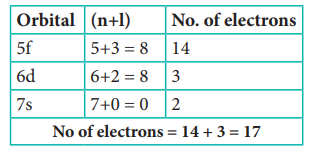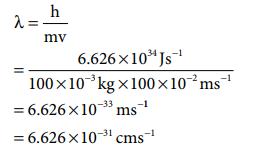Home | | Chemistry 11th std | Choose the best answer: Chemistry: Quantum Mechanical Model of Atom

# Choose the best answer: Chemistry: Quantum Mechanical Model of Atom

Chemistry : Quantum Mechanical Model of Atom : Choose the best answer

Quantum Mechanical Model of Atom

1. Electronic configuration of species M2+ is 1s2 2s2 2p6 3s2 3p6 3d6 and its atomic weight is 56. The number of neutrons in the nucleus of species M is

a) 26

b) 22

c) 30

d) 24

Solution:

M2+ : 1s2 2s2 2p6 3s2 3p6 3d6

M : 1s2 2s2 2p6 3s2 3p6 3d8

Atomic number = 26

Mass number =56

No. of neutrons = 56 -26 = 30

2. The energy of light of wavelength 45 nm is

a) 6.67 ├Ś 1015J

b) 6.67 ├Ś 1011J

c) 4.42 ├Ś 10ŌĆō18J

d) 4.42 ├Ś 10ŌĆō15J

Solution:

E = h╬Į = hc/╬╗

=6.626 ├Ś 10-34 J s ├Ś 3 ├Ś108 m s- / 45 ├Ś10-9 m

= 4 .42 ├Ś10-18 J

3. The energies E1 and E2 of two radiations are 25 eV and 50 eV respectively. The relation between their wavelengths ie ╬╗1 and ╬╗2 will be

a) ╬╗1/╬╗2 = 1

b) ╬╗1 = 2╬╗2

c) ╬╗1 =  ŌłÜ(25 ├Ś 50 ╬╗2)

d) 2╬╗1 = ╬╗2

Solution:4.  Splitting of spectral lines in an electric field is called

a) Zeeman effect

b) Shielding effect

c) Compton effect

d) Stark effect

Solution:

Spliting of spectral lines in magnetic field is called Zeeman effect and spliting of spectral lines in electric field, is called Stark effect.

5. Based on equation E = ŌĆō 2.178 ├Ś 10ŌĆō18 J (z2/n2) certain conclusions are written. Which of them is not correct ?

a) Equation can be used to calculate the change in energy when the electron changes orbit

b) For n = 1, the electron has a more negative energy than it does for n = 6 which means that the electron is more loosely bound in the smallest allowed orbit

c) The negative sign in equation simply means that the energy of electron bound to the nucleus is lower than it would be if the electrons were at the infinite distance from the nucleus.

d) Larger the value of n, the larger is the orbit radius.

Answer: b) For n = 1, the electron has a more negative energy than it does for n = 6 which means that the electron is more loosely bound in the smallest allowed orbit

Solution:

Correct statement: For n=1, the electron has more negative energy than it does for n=6 which means that the electron is strongly bound in the smallest allowed orbit.

6. According to the Bohr Theory, which of the following transitions in the hydrogen atom will give rise to the least energetic photon ?

a) n = 6 to n = 1

b) n = 5 to n = 4

c) n = 5 to n = 3

d) n = 6 to n = 5

Answer: d) n = 6 to n = 5

Solution:

n = 6 to n=5

E6 = -13.6 / 62 ;

E5 = - 13.6 / 52

E6 - E5 = (-13.6 /62) - (-13.6/52)

= 0.166 eV atom-1

E5 - E4 = (-13.6 /52) - (-13.6/42)

= 0.306 eV atom-1

7. Assertion : The spectrum of He+ is expected to be similar to that of hydrogen

Reason : He+ is also one electron system.

(a) If both assertion and reason are true and reason is the correct explanation of assertion.

(b) If both assertion and reason are true but reason is not the correct explanation of assertion.

(c) If assertion is true but reason is false

(d) If both assertion and reason are false

Answer: (a) If both assertion and reason are true and reason is the correct explanation of assertion.

8. Which of the following pairs of d-orbitals will have electron density along the axes ?

a) dz2, dxz

b) dxz, dyz

c) dz2, dx2-y2

d) dxy, dx2-y2

9. Two electrons occupying the same orbital are distinguished by

a) azimuthal quantum number

b) spin quantum number

c) magnetic quantum number

d) orbital quantum number

Solution:

Spin quantum number

For the first electron ms = +┬Į

For the second electron ms = -┬Į

10. The electronic configuration of Eu (Atomic no. 63) Gd (Atomic no. 64) and Tb (Atomic no. 65) are

a) [Xe] 4f6 5d1 6s2, [Xe] 4f7 5d1 6s2 and [Xe] 4f8 5d1 6s2

b) [Xe] 4f7 , 6s2, [Xe] 4f7 5d1 6s2 and [Xe] 4f9 6s2

c) [Xe] 4f7 , 6s2, [Xe] 4f8 6s2 and [Xe] 4f8 5d1 6s2

d) [Xe] 4f6 5d1 6s2, [Xe] 4f7 5d1 6s2 and [Xe] 4f9 6s2

Answer: b) [Xe] 4f7 , 6s2, [Xe] 4f7 5d1 6s2 and [Xe] 4f9 6s2

Solution:

Eu : [Xe] 4f7, 5d0, 6s2

Gd : [Xe] 4f7, 5d1, 6s2

Tb: [Xe] 4f9, 5d0, 6s2

11. The maximum number of electrons in a sub shell is given by the expression

a) 2n2

b) 2l + 1

c) 4l + 2

d) none of these

Solution:

2(2l+1) = 4l+2

12. For d-electron, the orbital angular momentum is

a) ŌłÜ(2h)/2ŽĆ

b) ŌłÜ(2)h/2ŽĆ

c) ŌłÜ(2x4) h/2ŽĆ

d) ŌłÜ(6)h/2ŽĆ

Solution:

Orbital angular momentum = ŌłÜ(l(l+1) h/2ŽĆ

For d orbital = ŌłÜ(2 x 3) h/2ŽĆ = ŌłÜ6 h/2ŽĆ

13) What is the maximum numbers of electrons that can be associated with the following set of quantum numbers ? n = 3, l = 1 and m = ŌĆō1

a) 4

b) 6

c) 2

d) = 10

Solution:

n = 3; l=1; m = -1

either 3px or 3py

i.e., Maximum two electrons can be accomodated either in 3px or in 3py

14. Assertion : Number of radial and angular nodes for 3p orbital are 1, 1 respectively.

Reason : Number of radial and angular nodes depends only on principal quantum num-ber.

a) both assertion and reason are true and reason is the correct explanation of as-sertion.

b) both assertion and reason are true but reason is not the correct explanation of assertion.

c) assertion is true but reason is false

d) both assertion and reason are false

Answer: c) assertion is true but reason is false

Solution:

No. of radial node = n-l-1

No. of angular node = l

for 3p orbital

No. of angular node = l = 1

No. of radial node = n-l-1 = 3-1-1 = 1

15. The total number of orbitals associated with the principal quantum number n = 3 is

a) 9

b) 8

c) 5

d) 7

Solution:

n = 3; l = 0; ml = 0 - one s orbital

n = 3; l = 1; ml = -1, 0, 1 - three p orbitals

n = 3; l = 2; ml = -2, -1, 0, 1, 2 - five d Orbitals

Overall nine orbitals are possible.

16. If n = 6, the correct sequence for filling of electrons will be,

a) ns ŌåÆ (n ŌĆō 2) f ŌåÆ (n ŌĆō 1)d ŌåÆ np

b) ns ŌåÆ (n ŌĆō 1) d ŌåÆ (n ŌĆō 2) f ŌåÆ np

c) ns ŌåÆ (n ŌĆō 2) f ŌåÆ np ŌåÆ (n ŌĆō 1) d

d) none of these are correct

Answer: a) ns ŌåÆ (n ŌĆō 2) f ŌåÆ (n ŌĆō 1)d ŌåÆ np

Solution:

n = 6

According Aufbau principle,

6s ŌåÆ4f ŌåÆ5d ŌåÆ6p

ns ŌåÆ (n-1)f ŌåÆ(n-2)d ŌåÆ np

17. Consider the following sets of quantum numbers :Which of the following sets of quantum number is not possible ?

a) (i), (ii), (iii) and (iv)

b) (ii), (iv) and (v)

c) and (iii)

d) (ii), (iii) and (iv)

Answer: b) (ii), (iv) and (v)

Solution:

(ii) l can have the values from 0 to n-1

n=2; possible 'l' values are 0, 1 hence = 2 is not possible.

(iv)for l=0; m=-1 not possible

(v)for n=3 l = 4 and m = 3 not possible

18. How many electrons in an atom with atomic number 105 can have (n + l) = 8 ?

a) 30

b) 17

c) 15

d) unpredictable

Solution:

n+l = 8

Electronic configuration of atom with atomic number 105 is [Rn] 5f14 6d3 7s219. Electron density in the yz plane of 3dx2 ŌłÆy2   orbital is

a) zero

b) 0.50

c) 0.75

d) 0.90

Solution:

Option (a) - Zero (Refer to Figure 2.9)

20. If uncertainty in position and momentum are equal, then minimum uncertainty in velocity isSolution:21. A macroscopic particle of mass 100 g and moving at a velocity of 100 cm sŌĆō1 will have a de Broglie wavelength of

a) 6.6 ├Ś 10ŌĆō29 cm

b) 6.6 ├Ś 10ŌĆō30 cm

c) 6.6 ├Ś 10ŌĆō31 cm

d) 6.6 ├Ś 10ŌĆō32 cm

Answer: c) 6.6 ├Ś 10ŌĆō31 cm

Solution:

m = 100 g = 100 x 10-3 kg

v= 100 cm s-1 = 100 x 10-2 m s-1

╬╗ =h/mv22) The ratio of de Broglie wavelengths of a deuterium atom to that of an ╬▒ - particle, when the velocity of the former is five times greater than that of later, is

a) 4

b) 0.2

c) 2.5

d) 0.4

23) The energy of an electron in the 3rd orbit of hydrogen atom is ŌĆōE. The energy of an electron in the first orbit will be

a) ŌĆō3E

b) ŌłÆE/3

c) ŌłÆE/9

d) ŌĆō9E

Solution:24) Time independent Schnodinger wave equation isAnswer: (a) HŌł¦ Žł = EŽł

25. Which of the following does not represent the mathematical expression for the Heisenberg uncertainty principle ?

a) Ōłåx.Ōłåp Ōēźh/4ŽĆ

b) Ōłåx.Ōłåv Ōēźh/4ŽĆm

c) ŌłåE.Ōłåt Ōēźh/4ŽĆ

d) ŌłåE.Ōłåx Ōēźh/4ŽĆ